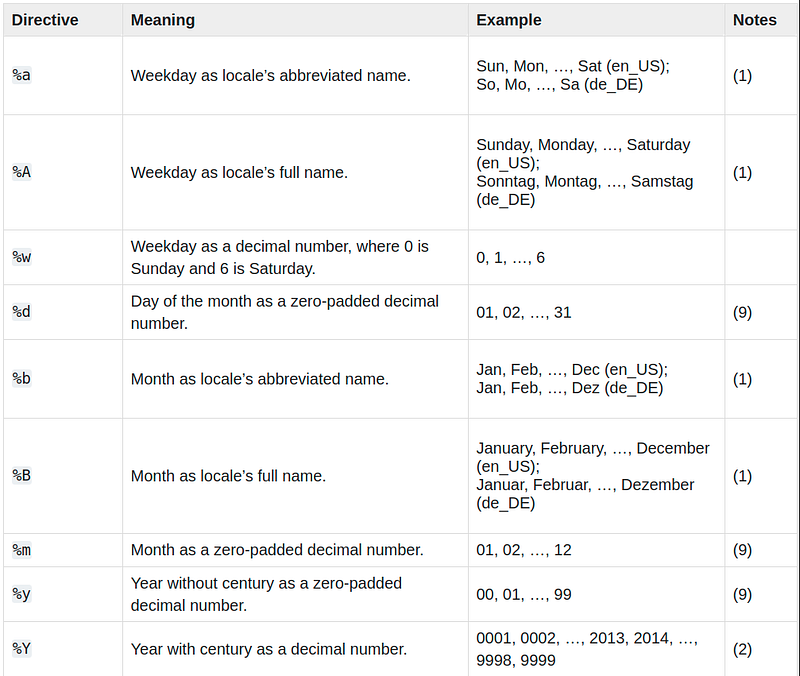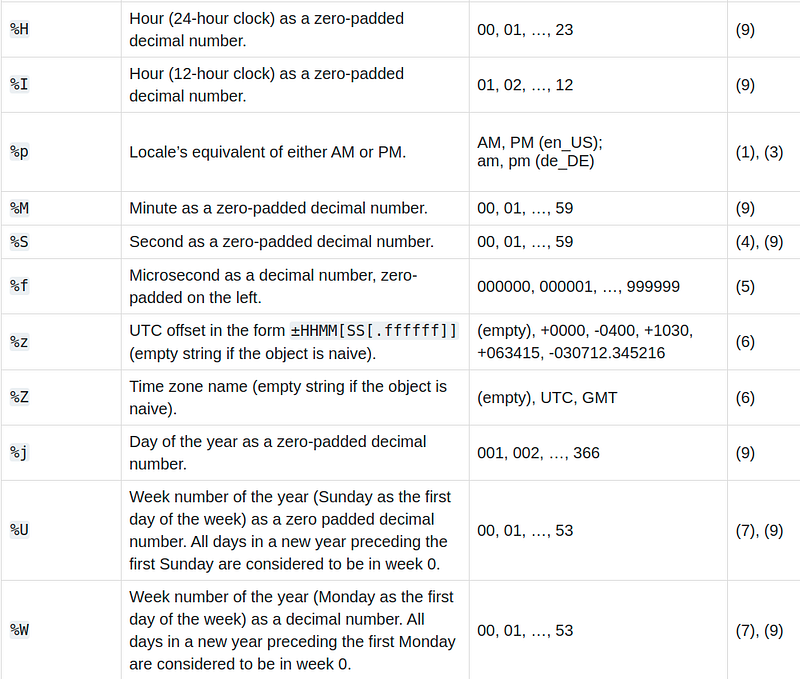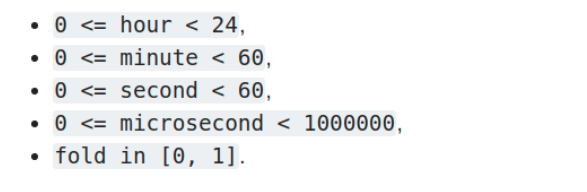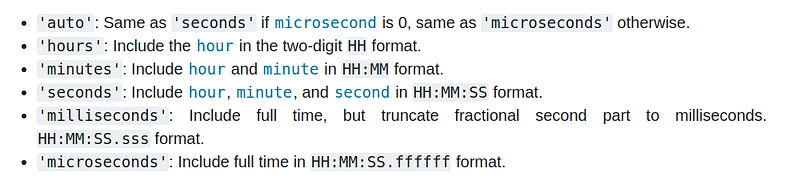Daniel Morales_

Maker - Data Scientist - Ruby on Rails Fullstack Developer

@daniel_moralesp

2020-12-18 18:02:21 UTC

## How to Use Python Datetimes Correctly

Datetime is basically a python object that represents a point in time, like years, days, seconds, milliseconds. This is very useful to create our programs.

The datetime module provides classes to manipulate dates and times in a simple and complex way. While date and time arithmetic is supported, the application focuses on the efficient extraction of attributes for formatting and manipulating output

Let’s import the Python module

In :

`from datetime import datetime`
Creating a date using year, month, and day as arguments.

datetime(year, month, day hour, minute, seconds)

In :

`birthday = datetime(1994, 2, 15, 4, 25, 12)`
Now once we’ve created the object and assigned to the variable called birthday, we can access to each date format just like this

In :

`birthday`
Out:

`datetime.datetime(1994, 2, 15, 4, 25, 12)`
In :

`birthday.year`
Out:

`1994`
In :

`birthday.month`
Out:

`2`
In :

`birthday.day`
Out:

`15`
As you can see, it’s very easy to create a date using this module. Now we can do other interesting things, like:

In :

`birthday.weekday()`
Out:

`1`
This means that the birthday was a Monday, because days are in this format (0-6), or what is the same indexed as a list (beginning with zero).

But what if I want to know what is the current datetime? In that case, we can use datetime.now(), Go ahead and write down this into next cell

In :

`datetime.now()`
Out:

`datetime.datetime(2020, 11, 17, 11, 32, 11, 992169)`
Ok, that’s interesting. What if you run that command again? Go ahead and see the difference

In :

`datetime.now()`
Out:

`datetime.datetime(2020, 11, 17, 11, 33, 36, 433919)`
As you can see the output is now different, because time changed. Great! Now you can ask, how do I calculate the time from one date to another? That’s called time tracking, let’s see how it works

In :

```# time tracking operation
datetime(2018, 1, 1) - datetime(2017, 1, 1)```
Out:

`datetime.timedelta(365)`
In :

`datetime(2018, 1, 1) - datetime(2017, 1, 12)`
Out:

`datetime.timedelta(354)`
You can see, how easy it is, we can run arithmetic operations between dates, which is great! But what if now you want to know how much time has passed from a given date to today, at this very moment? How do you think that can be done? Think about it for a moment!

In :

`datetime.now() - datetime(2020, 1, 1)`
Out:

`datetime.timedelta(321, 41994, 571469)`
Excellent now we use the .now() method and subtract the date we want to calculate. Easy!

Using strptime

This method will help us to transform dates that are given in strings to a datetime format, which is quite useful!

Let’s see it in action:

In :

`parsed_date = datetime.strptime('Nov 15, 2020', '%b %d, %Y')`
In :

`parsed_date`
Out:

`datetime.datetime(2020, 11, 15, 0, 0)`
In :

`type(parsed_date)`
Out:

`datetime.datetime`
As we see, we have passed two parameters to the strptime method, the first has been the string of the date, and the second the "directive" in which we want to make the conversion. To see all the available "directives", go to the following link:Image by pythonImage by python

We already have parsed our date in the parsed_date variable, now let's start making calls to the methods it contains.

In :

`parsed_date.month`
Out:

`11`
In :

`parsed_date.year`
Out:

`2020`
Using strftime

All right, now let’s do the opposite operation, passing a datetime type as a parameter to the strftime function and converting it to a string. We do it like this:

In :

`date_string = datetime.strftime(datetime.now(), '%b %d, %Y')`
In :

`date_string`
Out:

`'Nov 17, 2020'`
As you can see, we pass datetime.now() as the first argument and then the directives of the formats in which we want the output. Really simple!

Time object

A time object represents a time of day (local), independent of any particular day, and subject to adjustment through a tzinfo object.

All arguments are optional. tzinfo can be None, or an instance of a tzinfo subclass. The rest of the arguments can be integers, in the following ranges:Image by Author

If an argument is given outside these ranges, the Value-Error is raised.

All default values are 0 except tzinfo, which defaults to None. Time to play with this object!

In :

`from datetime import time`
In :

`my_time = time(hour=12, minute=34, second=56, microsecond=123456)`
In :

`my_time`
Out:

`datetime.time(12, 34, 56, 123456)`
As we can see it will give us a time object as a result. However, it has a not very “friendly” format. With the time object we can use the isoformat

In :

`my_time.isoformat(timespec='minutes')`
Out:

`'12:34'`
In :

`my_time.isoformat(timespec='microseconds')`
Out:

`'12:34:56.123456'`
In :

`my_time.isoformat(timespec='auto')`
Out:

`'12:34:56.123456'`
In :

`my_time.isoformat()`
Out:

`'12:34:56.123456'`
We can see that there are several iso formats to display the time. We use different formats, and the default one is auto, which we can use without passing a parameter explicitly. These are the possible fromatos to useImage by Author

timedelta object

A timedelta object represents a duration, the difference between two dates or times, which is quite useful! Let's look how it works. First we need to importa timedelta and then we need to call the different built-in functions

In :

`from datetime import timedelta`
In :

`year = timedelta(days=365)`
In :

`year`
Out:

`datetime.timedelta(365)`
In :

`year.total_seconds()`
Out:

`31536000.0`
In :

`ten_years = 10 * year`
In :

`ten_years.total_seconds()`
Out:

`315360000.0`
We’ve passed the parameter days = 365 to timedelta and then called two functions. One of them returns the total seconds that 365 days have.An the other one creates 10 years.

Let’s make another calculations

In :

```another_year = timedelta(weeks=40, days=84, hours=23,
minutes=50, seconds=600)  # adds up to 365 days```
In :

`another_year`
Out:

`datetime.timedelta(365)`
In :

`year == another_year`
Out:

`True`
We have now done a boolean operation, where we ask if one timedelta is the same as another. For which we get a True.

Naive & Aware methods

There are two types of date and time objects: “naive” & “aware”.

An “aware” object has sufficient knowledge of the applicable algorithmic and political time settings, such as time zone and daylight savings information, to be able to position itself in relation to other “aware” objects.

An “aware” object is used to represent a specific moment in time that is not open to interpretation. Ignore Relativity

A “naïve” object does not contain enough information to place itself unambiguously in relation to other date/time objects

Whether a “ship” object represents Coordinated Universal Time (UTC), local time or the time of some other time zone depends purely on the program, just as it depends on the program whether a given number represents meters, miles or mass

The “naive” objects are easy to understand and work with, at the cost of ignoring some aspects of reality.

This finally serves us to work time zones, and time changes (depending on summer-winter) and American zones as for example EST or EDT

Supporting time zones at deeper levels of detail depends on the application.

The rules for time adjustment worldwide are more political than rational, change frequently, and there is no standard suitable for every application other than UTC

Objects of this type are immutable.

Objects of the date type are always naive.